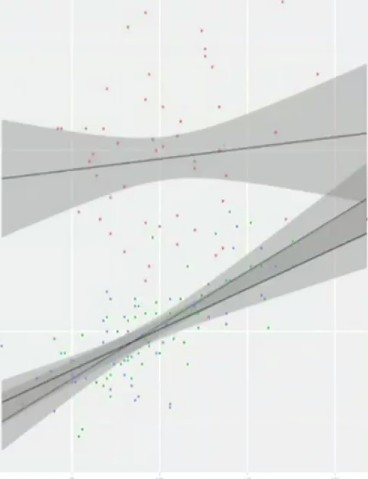# Statistics - Centering Continous Predictors

By putting all scores of a variable in a deviation form, the average for this variable will be equal to zero. It's called centering. To center means to put in deviation form.

To center a variable, take the individual score and subtract the mean.

Centering is essential when doing a moderation analysis, but it's helpful in any case.

Centring is changing the regression constant and the regression constant only. It doesn't change for example :

There is no data transformation. It's a really simple step that's basically just changing the scales on the graph.

If all values were z-scores, it would not be necessary to center the predictor variables in a multiple regression analysis because the mean is already 0.

## Reasons

### Conceptual

The main conceptual reason to center predictor variables in multiple regression is to make the regression constant more meaningful

In a regression analysis, the intercept or the regression coefficient $B_0$ is the predicted score on Y when all predictors are zero.

The regression constants didn't make any sense in a lot of example because it doesn't make any sense to get zero for all others predictors. It gives a meaningless regression constant $B_0$ .

### Statistical

#### Multicolinearity

The predictors, X and Z, can become highly correlated with the product, (X*Z)

When two predictor variables in a GLM are so highly correlated that they are essentially redundant and it becomes difficult to estimate B values associated with each predictor.

The regression coefficients, the slopes, or, the B values represent the unique variants explained in the outcome by each predictor.

If two predictors are basically redundant then it's going to be very difficult to figure out what unique variance either one of them explains and why.

You will run into very difficult mathematical computation problems in the matrix algebra (the GLM is just going to crash) if you have two predictors that are very strongly correlated (basically redundant).

##### How much of a correlation is bad and represents multicolineraity

There's no hard fast rule of thumb, the answer is typically anything above 0.8.

If you have two predictors in a Russian model that are correlated over 0.8 they're pretty redundant. They might cause a problem computationally in the matrix algebra, so you might want to pull one of them out of the regression model.

Recommended PagesStatistics - Deviation Score (for one observation)

The deviation score is the difference between two scores as : a value and an other value (usually the predicted score: mean, ...) See also: for a sample, for a measurement, (??) ...Statistics - Intercept - Regression (coefficient|constant)

In a linear equation, the intercept is the point at which the line crosses the y-axis, otherwise known as the y-intercept In the below linear equation, b below is the intercept: In a regression analysis,...Statistics - Moderator Variable (Z) - Moderation

A moderation analysis is a multiple regression analysis. The main reason to run a moderation analysis is to demonstrate how a third variable (Z) changes the correlation between two variables (X and Y)....